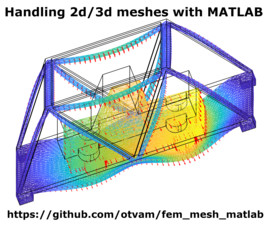# Application ExchangeFilename Size
fem_mesh_matlab-master.zip 7.3 MB

## MATLAB Toolbox for Handling 2D and 3D FEM Meshes

Thomas Guillod, Personnal account

This MATLAB toolbox offers numerous functionalities for handling 2d and 3d meshes:
* Parse geometry (edge, surface, and volume) - 'extract_geom.m'
* Plot geometry (edge, surface, volume, normal vector, and tangential vector) - 'plot_geom.m'
* Assign variables to the vertices of the meshes - 'extract_data.m'
* Plot variables (scalar variable and vector field) - 'plot_data.m'
* Integrate variables on the meshes (scalar variable and vector field) - 'integrate_data.m'
* Interpolate variables on the meshes - 'interp_data.m'

The toolbox can be used for the following tasks:
* Processing finite element method (FEM) results (e.g. COMSOL, ANSYS, OpenFOAM)
* Handling geographic information system (GIS) data (e.g. digital elevation model)
* Handling additive manufacturing data (e.g. 3d printing)